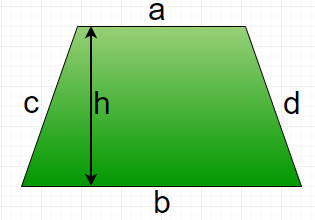# Program to calculate area and perimeter of Trapezium

A trapezium is a quadrilateral with at least one pair of parallel sides, other two sides may not be parallel. The parallel sides are called the bases of the trapezium and the other two sides are called it’s legs. The perpendicular distance between parallel sides is called height of trapezium.Formula :

```Area of Trapezium : 0.5 * (a + b) * h
Perimeter of Trapezium : a + b + c + d
```

Examples :

```Input :  a = 5, b = 6, c = 4, d = 3, h = 8
Output : Area of Trapezium : 44
Perimeter of Trapezium : 18

Input :  a = 10, b = 15, c = 14, d = 11, h = 21
Output : Area of Trapezium: 262.5
Perimeter of Trapezium: 50
```

## Recommended: Please try your approach on {IDE} first, before moving on to the solution.

Below is the implementation of above formula :

## C++

 `// CPP program to find area ` `// and perimeter of trapezium ` `#include ` `using` `namespace` `std; ` ` `  `// Function to calculate Area of trapezium ` `float` `areaTrapezium(``float` `a, ``float` `b, ``float` `h) ` `{ ` `    ``return` `(1.0 / 2 * (a + b) * h); ` `} ` ` `  `// Function to calculate perimeter of trapezium ` `float` `perimeterTrapezium(``float` `a, ``float` `b, ``float` `c,  ` `                                           ``float` `d) ` `{ ` `    ``return` `(a + b + c + d); ` `} ` ` `  `// Driver function ` `int` `main() ` `{ ` `    ``float` `a = 5, b = 15, c = 11, d = 4, h = 20; ` `    ``cout << ``"Area of Trapezium = "` `<<  ` `                ``areaTrapezium(a, b, h) << endl; ` `    ``cout << ``"Perimeter of Trapezium = "` `<<  ` `                ``perimeterTrapezium(a, b, c, d); ` `    ``return` `0; ` `} `

## Java

 `// Java program to calculate area ` `// and perimeter  of Trapezium ` ` `  `public` `class` `GFG { ` `   `  `    ``// Function to calculate area of Trapezium ` `    ``public` `static` `float` `areaTrapezium (``float` `a, ` `                                ``float` `b, ``float` `h) ` `    ``{ ` `        ``return` `((a + b) * h) / ``2``; ` `    ``} ` `     `  `    ``// Function to perimeter of Trapezium ` `    ``public` `static` `float` `perimeterTrapezium (``float` `a, ` `                            ``float` `b, ``float` `c, ``float` `d) ` `    ``{ ` `        ``return` `(a + b + c + d); ` `    ``} ` ` `  `     `  ` `  `// Driver function ` `public` `static` `void` `main(String args[]) ` `{ ` ` `  `    ``// a, b, c, d are four sides of Trapezium  ` `    ``// and h is height between two parallel sides. ` `    ``float` `a = ``5``; ` `    ``float` `b = ``15``; ` `    ``float` `c = ``11``; ` `    ``float` `d = ``4``; ` `    ``float` `h = ``20``; ` ` `  `    ``// Printing value of  area. ` `    ``System.out.print(``"Area Of Trapezium : "``); ` `    ``System.out.println(areaTrapezium (a, b, h)); ` `     `  `    ``// Printing value of  Perimeter.  ` `    ``System.out.print(``"Perimeter Of Trapezium : "``); ` `    ``System.out.println(perimeterTrapezium (a, b, c, d)); ` `} ` ` `  `} ` ` `  `// This code is contributed by "akanshgupta" `

## Python3

 `# Python3 code to find area ` `# and perimeter of trapezium ` ` `  `# Function to calculate  ` `# Area of trapezium ` `def` `areaTrapezium (a, b, h): ` `    ``return` `(``1.0` `/` `2` `*` `(a ``+` `b) ``*` `h) ` ` `  `# Function to calculate  ` `# perimeter of trapezium ` `def` `perimeterTrapezium (a, b, c, d): ` `    ``return` `(a ``+` `b ``+` `c ``+` `d) ` ` `  `# Driver function ` `a ``=` `5` `b ``=` `15` `c ``=` `11` `d ``=` `4` `h ``=` `20` `print``(``"Area of Trapezium ="``, ` `      ``areaTrapezium(a, b, h)) ` ` `  `print``(``"Perimeter of Trapezium ="``, ` `      ``perimeterTrapezium(a, b, c, d)) ` ` `  `# This code is contributed by "Sharad_Bhardwaj" `

## C#

 `// C# program to calculate area ` `// and perimeter of Trapezium ` `using` `System; ` ` `  `class` `GFG { ` ` `  `    ``// Function to calculate area of Trapezium ` `    ``public` `static` `float` `areaTrapezium (``float` `a, ` `                                 ``float` `b, ``float` `h) ` `    ``{ ` `        ``return` `((a + b) * h) / 2; ` `    ``} ` `     `  `    ``// Function to perimeter of Trapezium ` `    ``public` `static` `float` `perimeterTrapezium (``float` `a, ` `                            ``float` `b, ``float` `c, ``float` `d) ` `    ``{ ` `        ``return` `(a + b + c + d); ` `    ``} ` ` `  `     `  ` `  `    ``// Driver function ` `    ``public` `static` `void` `Main() ` `    ``{ ` `     `  `        ``// a, b, c, d are four sides of Trapezium  ` `        ``// and h is height between two parallel sides. ` `        ``float` `a = 5; ` `        ``float` `b = 15; ` `        ``float` `c = 11; ` `        ``float` `d = 4; ` `        ``float` `h = 20; ` `     `  `        ``// Printing value of area. ` `        ``Console.Write(``"Area Of Trapezium : "``); ` `        ``Console.WriteLine(areaTrapezium (a, b, h)); ` `         `  `        ``// Printing value of Perimeter.  ` `        ``Console.Write(``"Perimeter Of Trapezium : "``); ` `        ``Console.WriteLine(perimeterTrapezium (a, b, c, d)); ` `    ``} ` ` `  `} ` ` `  `// This code is contributed by "vt_m" `

## PHP

 ` `

Output:

```Area of Trapezium = 200
Perimeter of Trapezium = 35
```

GeeksforGeeks has prepared a complete interview preparation course with premium videos, theory, practice problems, TA support and many more features. Please refer Placement 100 for details

My Personal Notes arrow_drop_upCheck out this Author's contributed articles.

If you like GeeksforGeeks and would like to contribute, you can also write an article using contribute.geeksforgeeks.org or mail your article to contribute@geeksforgeeks.org. See your article appearing on the GeeksforGeeks main page and help other Geeks.

Please Improve this article if you find anything incorrect by clicking on the "Improve Article" button below.

Improved By : vt_m